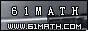Today is . WELCOME: huohai3983/44663  Home | Reg Login | All user | setHomePage | BookmarkHome | Math Test Online | Addition | Subtraction | Multiplication | Division | Time | Worksheet(JPG/PDF) | CAI | Flash Games |Recommendation articleArticle searchHome--maths--Third Grade--Add hours

How to add hours to a time:

• Add the number of hours to the hours portion of the time.
• If the number of hours is more than 12 do the following:
• Subtract 12 from the number of hours.
• If the time was AM change it to PM, if it was PM change it to AM.
• Repeat this process if the number of hours is still more than 12.
• If the number of hours is equal to 12 change AM to PM or PM to AM.

Example: What time is 16 hours after 9:00 AM?

• Add the hours together. (16 hours + 9 hours = 25 hours)
• If the number of hours is more than 12 do the following:
• Subtract 12 from the number of hours. (25 hours - 12 hours = 13 hours)
• Change the AM to PM.
• Repeat this process if the number of hours is still more than 12.
• Subtract 12 hours from 13 hours. Change the PM to AM.

#### What is the Sum of the times?

2006-11-18 22:40:56
Viewed 2472 times CloseGreatmathsites